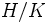# Transfer to an abelian group

## Definition

Let$G$ be a finite group and$H$ and$K$ be subgroups such that$K \triangleleft H$ and$H/K$ is abelian and$H$ has finite index in$G$. Let$T$ be a left transversal of$H$ in$G$. Then define the following mapping$V: G \to H/K$$V(x) = \prod_{t \in T} h_x^T(t)K$

here$h_x^T(t)$ is the unique element$h \in H$ such that$xt = t'h$ for some$t' \in T$.

We need to quotient out by$K$ so that the product on the right side is independent of the order of terms in the transversal.

## Facts

### Homomorphism

The transfer is a homomorphism of groups from$G$ to$H/K$.

### Independence of choice of transversal

The transfer map does not depend on the choice of transversal$T$.